# dy For a particular chemical reaction the rate (ghr) at which one of the reactants changes is proportional to the amoun...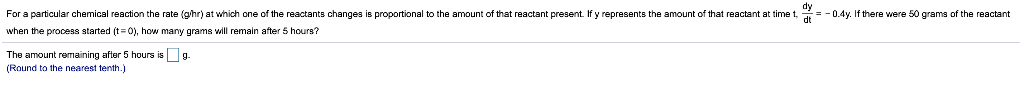dy For a particular chemical reaction the rate (ghr) at which one of the reactants changes is proportional to the amount of that reactant present If y represents the amount of that reactant at -0.4y. If there were 50 grams of the reactant dt when the process started (t-O), how many grams will remain after 5 hours? The amount remaining after 5 hours is9 (Round to the nearest tenth.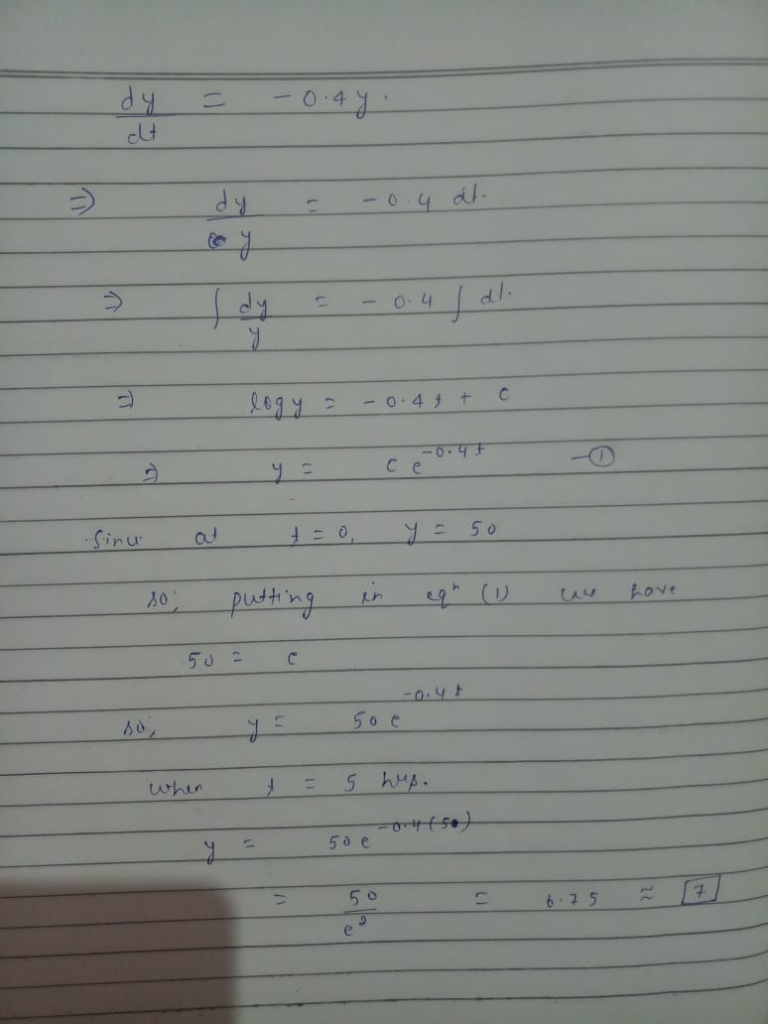##### Add Answer of: dy For a particular chemical reaction the rate (ghr) at which one of the reactants changes is proportional to the amoun...
Similar Homework Help Questions
• ### First Order Chemical Reactions

In some chemical reactions the rate at which the amount of asubstance changes with time is proportional to the amount present.For the cahnge of δ- glucono lactoneinto gluconic acid, forexample,dy/dt = - 0.6ywhen y is measured in grams and t is measured in hours. Ifthere are 100 grams of a δ- glucono lactone present when t=0,how many grams will be left after the firsthour?PLEASE SHOW WORK!!!!!!!THANKS!!!!!!!

• ### (1 point) Two chemicals A and B are combined to form a chemical C. The rate of the reaction is proportional to the prod...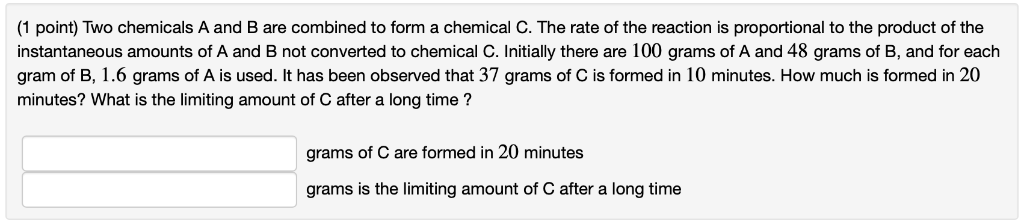(1 point) Two chemicals A and B are combined to form a chemical C. The rate of the reaction is proportional to the product of the instantaneous amounts of A and B not converted to chemical C. Initially there are 100 grams of A and 48 grams of B, and for each gram of B, 1.6 grams of A is used. It has been observed that 37 grams of C is formed in 10 minutes. How much is formed in...

• ### (1 point) Two chemicals A and B are combined to form a chemical C. The rate of the reaction is proportional to the prod...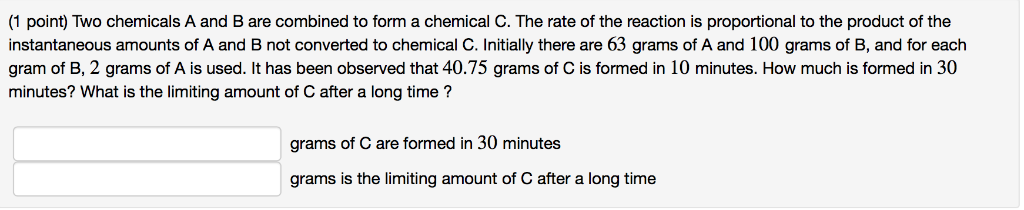(1 point) Two chemicals A and B are combined to form a chemical C. The rate of the reaction is proportional to the product of the instantaneous amounts of A and B not converted to chemical C. Initially there are 63 grams of A and 100 grams of B, and for each gram of B, 2 grams of A is used. It has been observed that 40.75 grams of C is formed in 10 minutes. How much is formed in...

• ### This question is about the rate of a chemical reaction between two reactants, one of which is in solutuion and one of which is solid?

which of the following changes would NOT cause the rate of chemical reactions to increase. increasing the concentration of the solutions heating the reaction mixture to a higher temperature using a larger volume of the solution, but keeping the concentration the same grinding the solid reactants so that it forms a a fine powder.

• ### According to the collision theory, which of the following statements is true? Which of the following would speed up the rate of a chemical reaction?

8. [06.04] According to the collision theory, which of the following statements is true? Products are formed from any orientation of the reactants. Molecules intentionally orientate themselves to produce the desired products. Reactant molecules must collide in a specific orientation for products to form. It is easy for molecules to react because they must simply collide. 9. [06.04] Which of the following would speed up the rate of a chemical reaction? Decreasing the temperature Increasing the concentration of reactants Decreasing...

• ### Please answer the following questions using exponential and logarithmic models. 4) A wooden artif...

Please answer the following questions using exponential and logarithmic models. 4) A wooden artifact from an archaeological dig contains 70 percent of the Carbon-14 that is present in living trees. To the nearest year, about how many years old is the artifact? (The half-life of Carbon-14 is 5730 years.) In years 5) A tumor is injected with 0.5 grams of Iodine-125, which has a decay rate of 1.15% per day. Write an exponential model representing the amount of Iodine-125 remaining...

• ### help!!! Problem Statement: How can concentration changes affect a chemical reaction? I, Data Collection: EXPERIMENT #...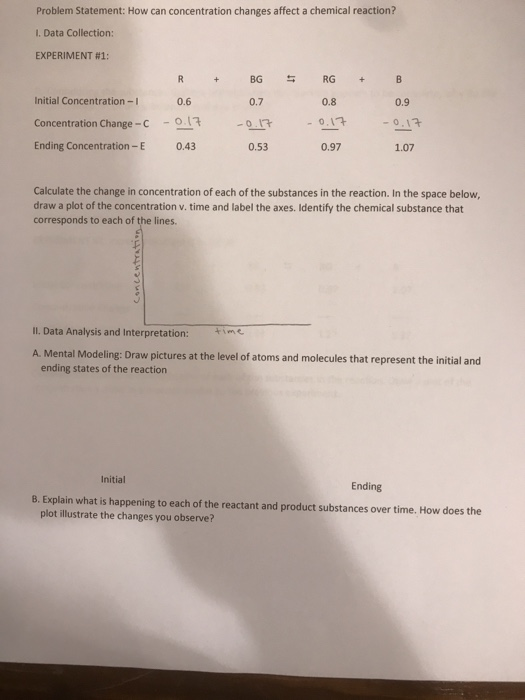help!!! Problem Statement: How can concentration changes affect a chemical reaction? I, Data Collection: EXPERIMENT # 1 : R BG RG B + + Initial Concentration-I 0.6 0.7 0.8 0.9 - 0.13 Concentration Change-C -0.17 - 0,17 0.17 Ending Concentration-E 0.43 0.53 0.97 1.07 Calculate the change in concentration of each of the substances in the reaction. In the space below, draw a plot of the concentration v. time and label the axes. Identify the chemical substance that corresponds to...

• ### need help with the boxed bullet point. writing the chemical reaction scheme for the experiment. Synthesis of an A...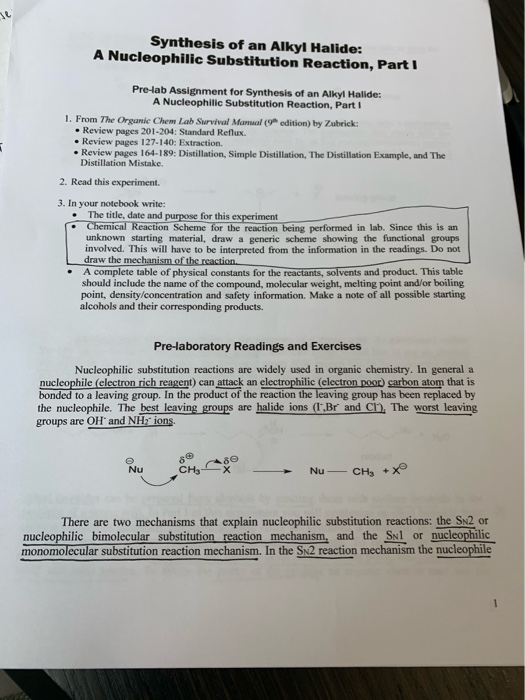need help with the boxed bullet point. writing the chemical reaction scheme for the experiment. Synthesis of an Alkyl Halide: A Nucleophilic Substitution Reaction, Part I Pre-lab Assignment for Synthesis of an Alkyl Halide: A Nucleophilic Substitution Reaction, Part 1 1. From The Organic Chem Lab Survival Manual ( edition) by Zubrick • Review pages 201-204: Standard Reflux. • Review pages 127-140: Extraction • Review pages 164-189: Distillation, Simple Distillation. The Distillation Example, and The Distillation Mistake. 2. Read this...

• ### just one example/demonstration! Data needed to be calculated is in highlighted in green boxes. And I...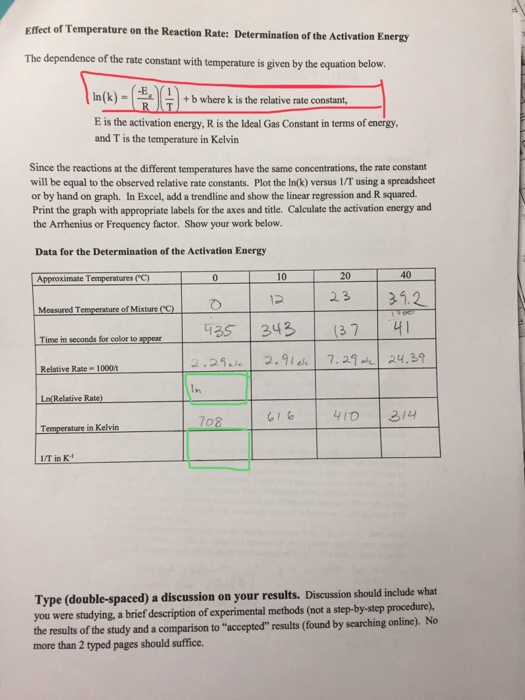just one example/demonstration! Data needed to be calculated is in highlighted in green boxes. And I highlighted in red an equation (not sure if thats what you use to calculate it) And ignore the lab instructions on completeing a graph!! I already know how to do that in excel, just curious how Ln (relative rate) and 1/T in K^-1 is calculated by hand* here is the rest of that lab leading up to the question as I know its typically...

• ### Grignard reagent THE GRIGNARD REAGENT-PREPARATION AND REACTION In this experiment, you will prepa...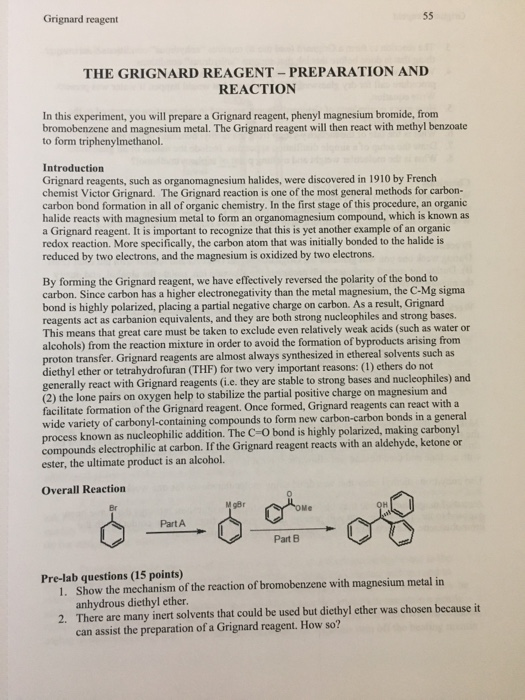i need help with the postlab questions please Grignard reagent THE GRIGNARD REAGENT-PREPARATION AND REACTION In this experiment, you will prepare a Grignard reagent, phenyl magnesium bromide, from bromobenzene and magnesium metal. The Grignard reagent will then react with methyl benzoate to form triphenylmethanol. Introduction Grignard reagents, such as organomagnesium halides, were discovered in 1910 by French chemist Victor Grignard. The Grignard reaction is one of the most general methods for carbon- carbon bond formation in all of organic chemistry....

Need Online Homework Help?